# Spherical Geometries

Learning objectives:

• Consider geometries on a sphere
library("dplyr")
library("ggplot2")
library("maps")
library("rnaturalearth")
library("rnaturalearthdata")
library("s2")
library("sf")

sessionInfo()
R version 4.2.2 (2022-10-31 ucrt)
Platform: x86_64-w64-mingw32/x64 (64-bit)
Running under: Windows 10 x64 (build 19044)

Matrix products: default

locale:
 LC_COLLATE=English_United States.utf8
 LC_CTYPE=English_United States.utf8
 LC_MONETARY=English_United States.utf8
 LC_NUMERIC=C
 LC_TIME=English_United States.utf8

attached base packages:
 stats     graphics  grDevices utils     datasets  methods   base

other attached packages:
 sf_1.0-12               s2_1.1.3                rnaturalearthdata_0.1.0
 rnaturalearth_0.3.2     maps_3.4.1              ggplot2_3.4.2
 dplyr_1.1.2

loaded via a namespace (and not attached):
 Rcpp_1.0.10        pillar_1.9.0       compiler_4.2.2     class_7.3-20
 tools_4.2.2        digest_0.6.31      lattice_0.20-45    jsonlite_1.8.4
 evaluate_0.21      lifecycle_1.0.3    tibble_3.2.1       gtable_0.3.3
 pkgconfig_2.0.3    rlang_1.1.0        cli_3.6.0          DBI_1.1.3
 rstudioapi_0.14    xfun_0.39          fastmap_1.1.1      e1071_1.7-13
 withr_2.5.0        httr_1.4.5         knitr_1.42         generics_0.1.3
 vctrs_0.6.1        htmlwidgets_1.6.2  classInt_0.4-9     grid_4.2.2
 tidyselect_1.2.0   glue_1.6.2         R6_2.5.1           fansi_1.0.4
 rmarkdown_2.21     sp_1.6-0           magrittr_2.0.3     units_0.8-2
 scales_1.2.1       htmltools_0.5.4    colorspace_2.1-0   KernSmooth_2.23-20
 utf8_1.2.3         proxy_0.4-27       wk_0.7.3           munsell_0.5.0     

## Straight Lines

1. How does the GeoJSON format (Butler et al. 2016) define “straight” lines between ellipsoidal coordinates (Section 3.1.1)? Using this definition of straight, how does LINESTRING(0 85,180 85) look like in an Arctic polar projection? How could this geometry be modified to have it cross the North Pole?
this_linestring <- st_linestring(matrix(c(0, 85, 180, 85), ncol = 2),
dim = "XY")
class(this_linestring)
 "XY"         "LINESTRING" "sfg"       
this_linestring |>
ggplot() +
geom_sf(color = "red", linewidth = 3) +
labs(title = "This Linestring",
subtitle = "Where is it?",
caption = "Spatial Data Science book club") +
theme_minimal()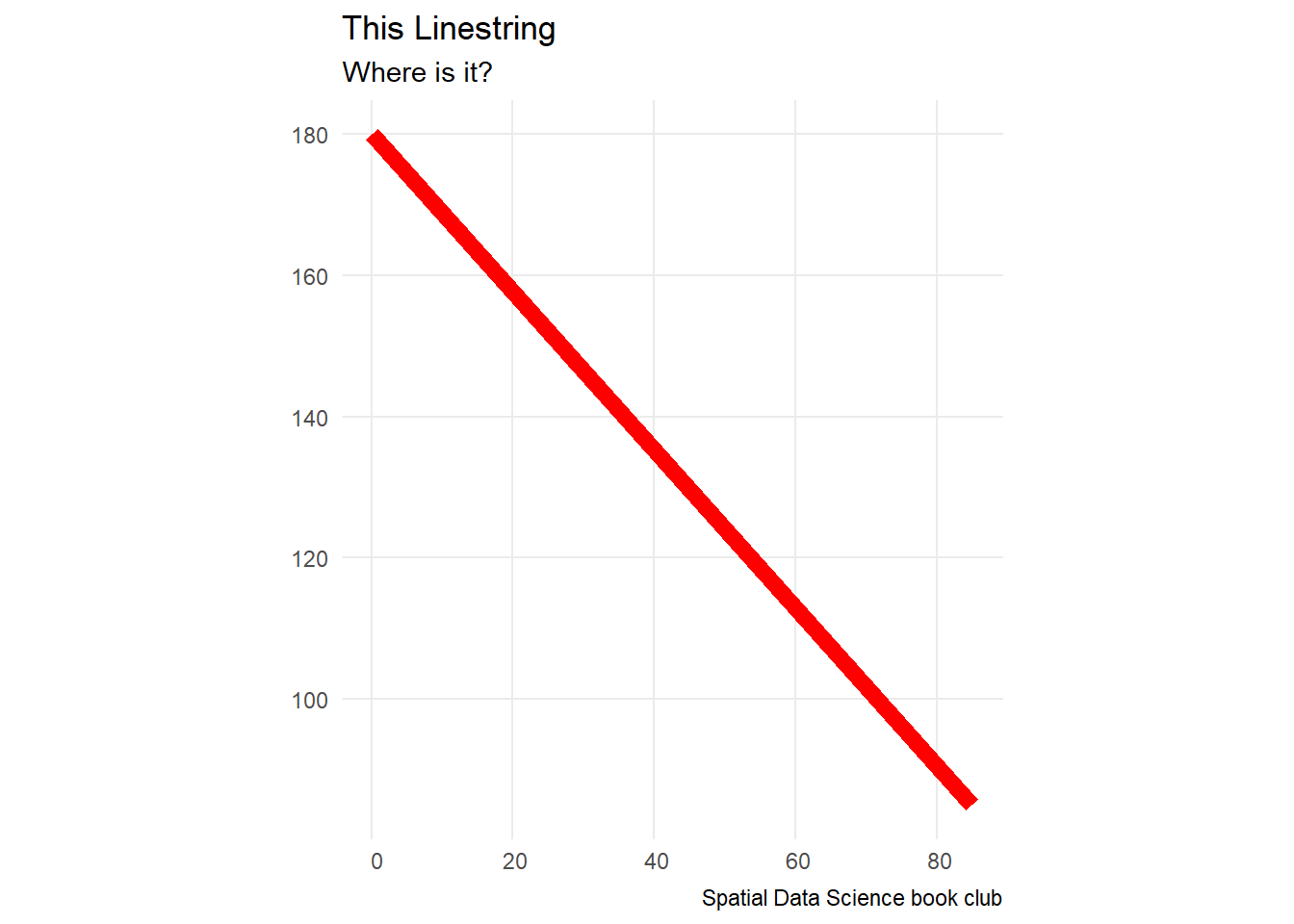# https://stackoverflow.com/questions/58919102/map-arctic-subarctic-regions-in-ggplot2-with-lambert-conformal-conic-projection
world <- ne_countries(scale = "medium", returnclass = "sf")
world_cropped <- world |>
st_make_valid() |>
st_crop(xmin = -180.0, xmax = 180.0, ymin = 45.0, ymax = 90.0)
Warning: attribute variables are assumed to be spatially constant throughout
all geometries
# st_sfc(this_linestring) <- st_crs(world_cropped)

# this_linestring_sf <- st_sf(this_linestring,
#                             st_sfc(this_linestring, crs = "EPSG:3995"))

ggplot(data = world_cropped) +
geom_sf() +
# geom_sf(data = this_linestring, color = 'red') +
coord_sf(crs =
"+proj=lcc +lat_1=50 +lat_2=70 +lat_0=40 +lon_0=-96 +x_0=0 +y_0=0 +datum=NAD83 +units=m +no_defs +ellps=GRS80 +towgs84=0,0,0")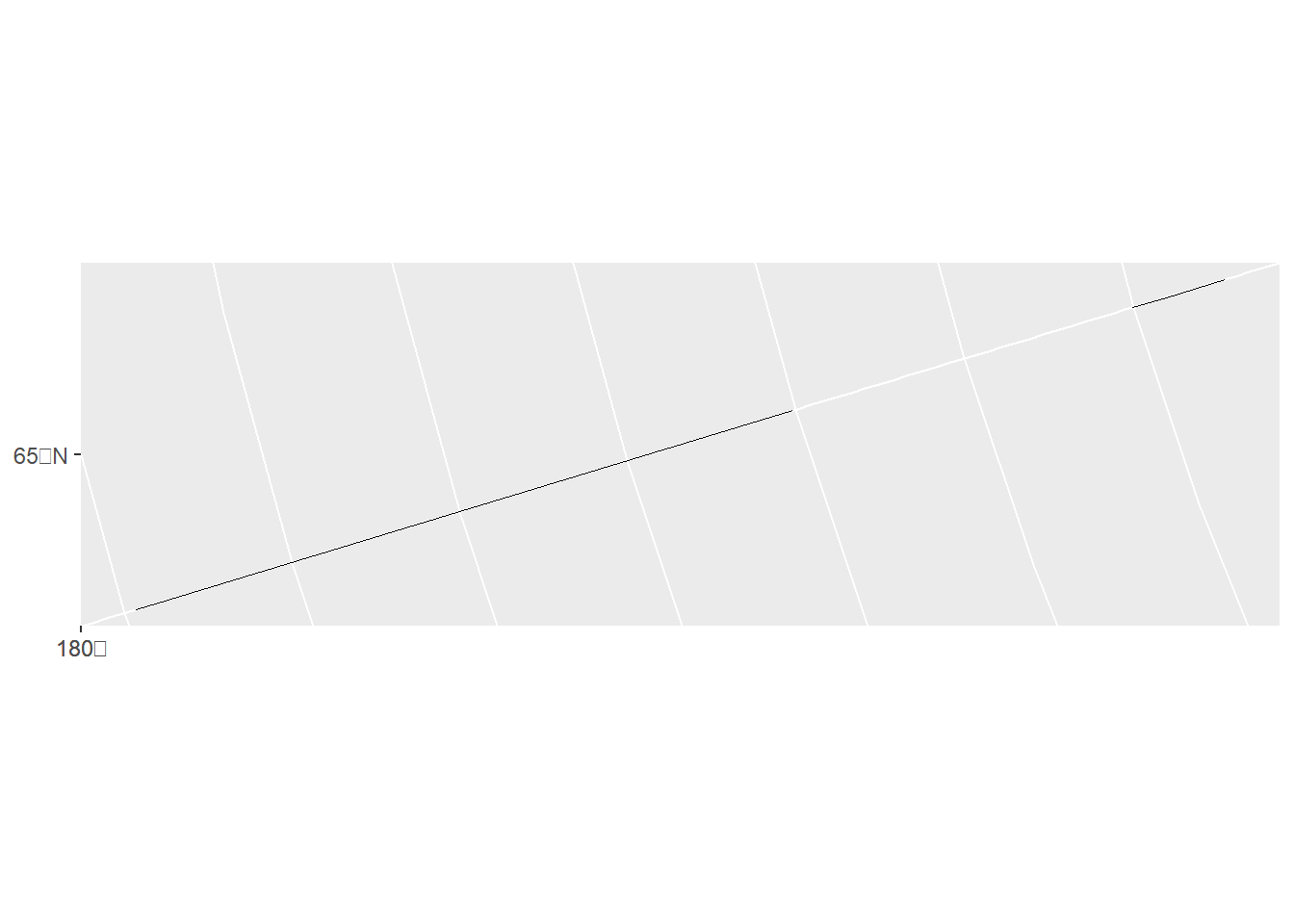## Ring Direction

For a typical polygon on $$S^2$$, how can you find out ring direction?

• A convention here is to define the inside as the left (or right) side of the polygon boundary when traversing its points in sequence. Reversal of the node order then switches inside and outside.

• Additional resource: ESRI: Polygon page

## Bounding Boxes

Antarctica_map <- map(fill = TRUE, plot = FALSE) |>
st_as_sf() |>
filter(ID == "Antarctica")

Antarctica_map |>
ggplot() +
geom_sf() +
labs(title = "Antarctica",
subtitle = "Think of the latitude",
caption = "Spatial Data Science book club")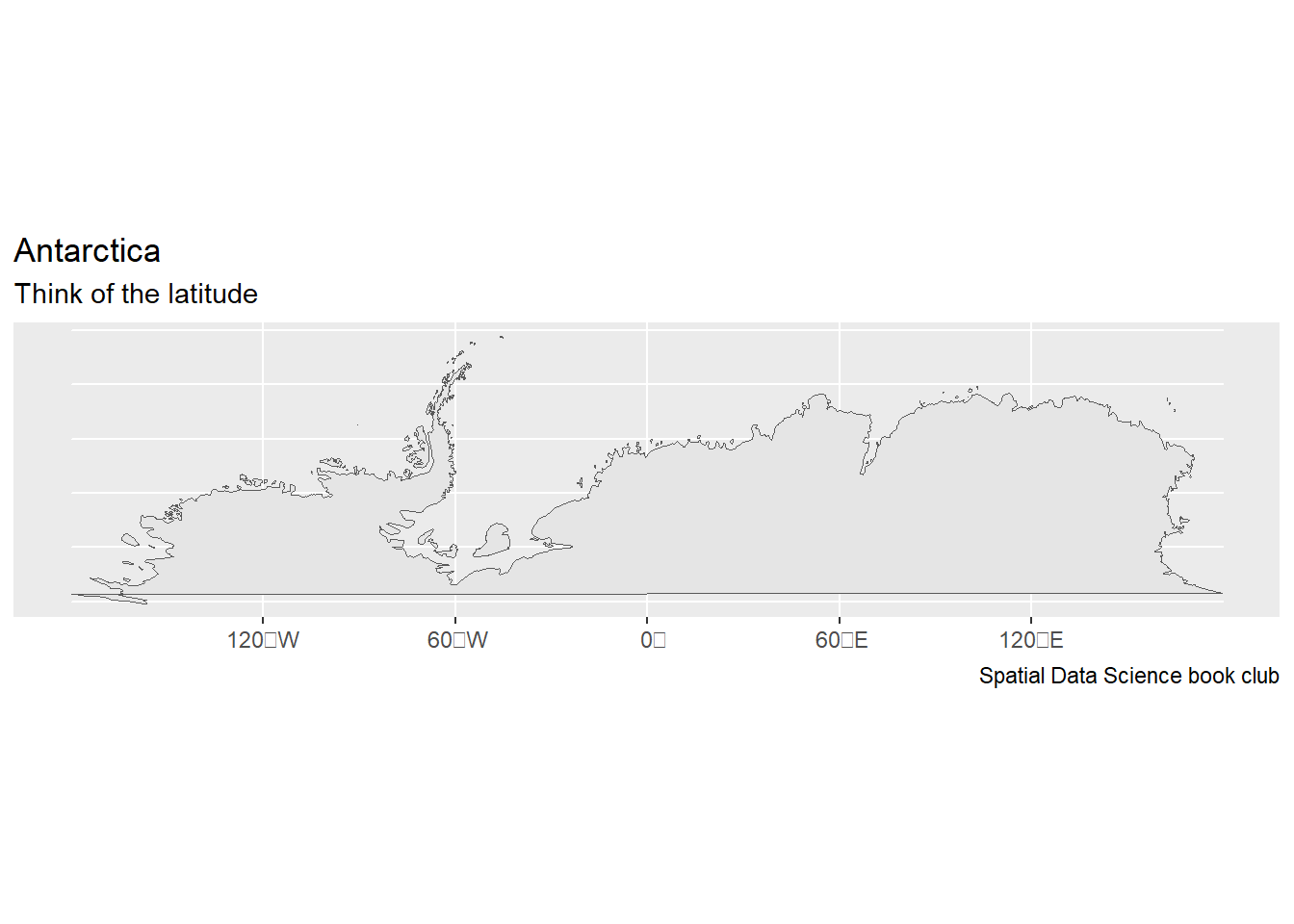st_bbox(Antarctica_map)
      xmin       ymin       xmax       ymax
-180.00000  -85.19218  179.62032  -60.52090 

which clearly does not contain the region (ymin being -90 and xmax 180).

Fiji_map <- map(fill = TRUE, plot = FALSE) |>
st_as_sf() |>
filter(ID == "Fiji")

Fiji_map |>
ggplot() +
geom_sf() +
labs(title = "Fiji",
subtitle = "Think of the longitude",
caption = "Spatial Data Science book club")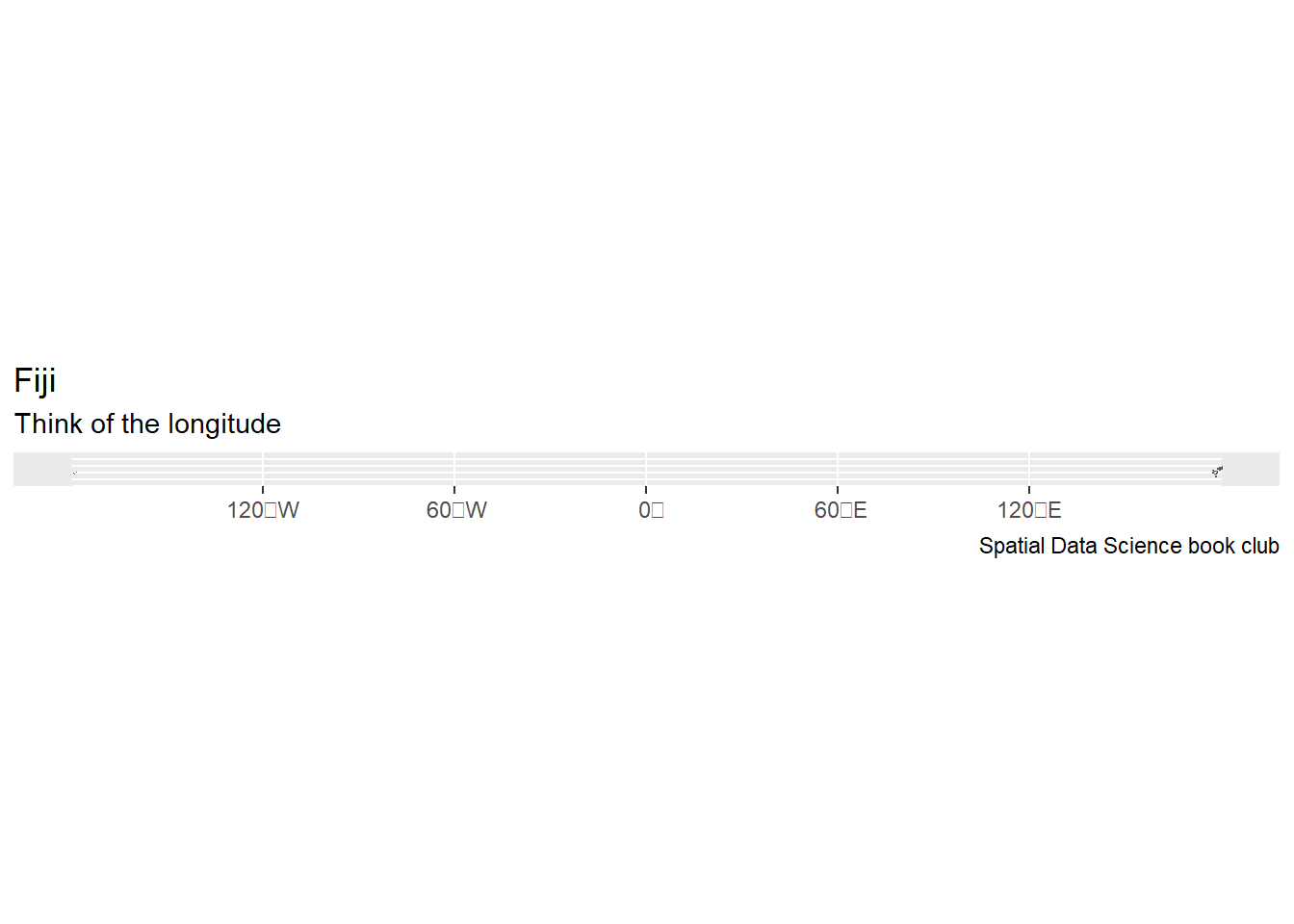st_bbox(Fiji_map)
      xmin       ymin       xmax       ymax
-179.86734  -21.70586  180.17769  -12.47695 

seems to span most of the Earth

### Spherical Coordinates

s2_bounds_cap(Antarctica_map)
  lng lat   angle
1   0 -90 29.4791
s2_bounds_rect(Antarctica_map)
  lng_lo lat_lo lng_hi   lat_hi
1   -180    -90    180 -60.5209
s2_bounds_rect(Fiji_map)
    lng_lo    lat_lo    lng_hi    lat_hi
1 174.5872 -21.70586 -178.2511 -12.47695

## Validity

Maps of Antarctica should probably display the South Pole. Do the following maps display the South Pole?

### Planar (Ellipsoidal Coordinates)

# maps package
m <- st_as_sf(map(fill=TRUE, plot=FALSE))
Antarctica_map_A <- m[m$ID == "Antarctica", ] st_geometry(Antarctica_map_A) |> ggplot() + geom_sf() + labs(title = "Antarctica", subtitle = "Think of the latitude", caption = "Spatial Data Science book club")sf::st_is_valid(Antarctica_map_A)  TRUE # Natural Earth package ne <- ne_countries(returnclass = "sf") Antarctica_map_B <- ne[ne$region_un == "Antarctica", "region_un"]
st_geometry(Antarctica_map_B) |>
ggplot() +
geom_sf() +
labs(title = "Antarctica",
subtitle = "Think of the latitude",
caption = "Spatial Data Science book club")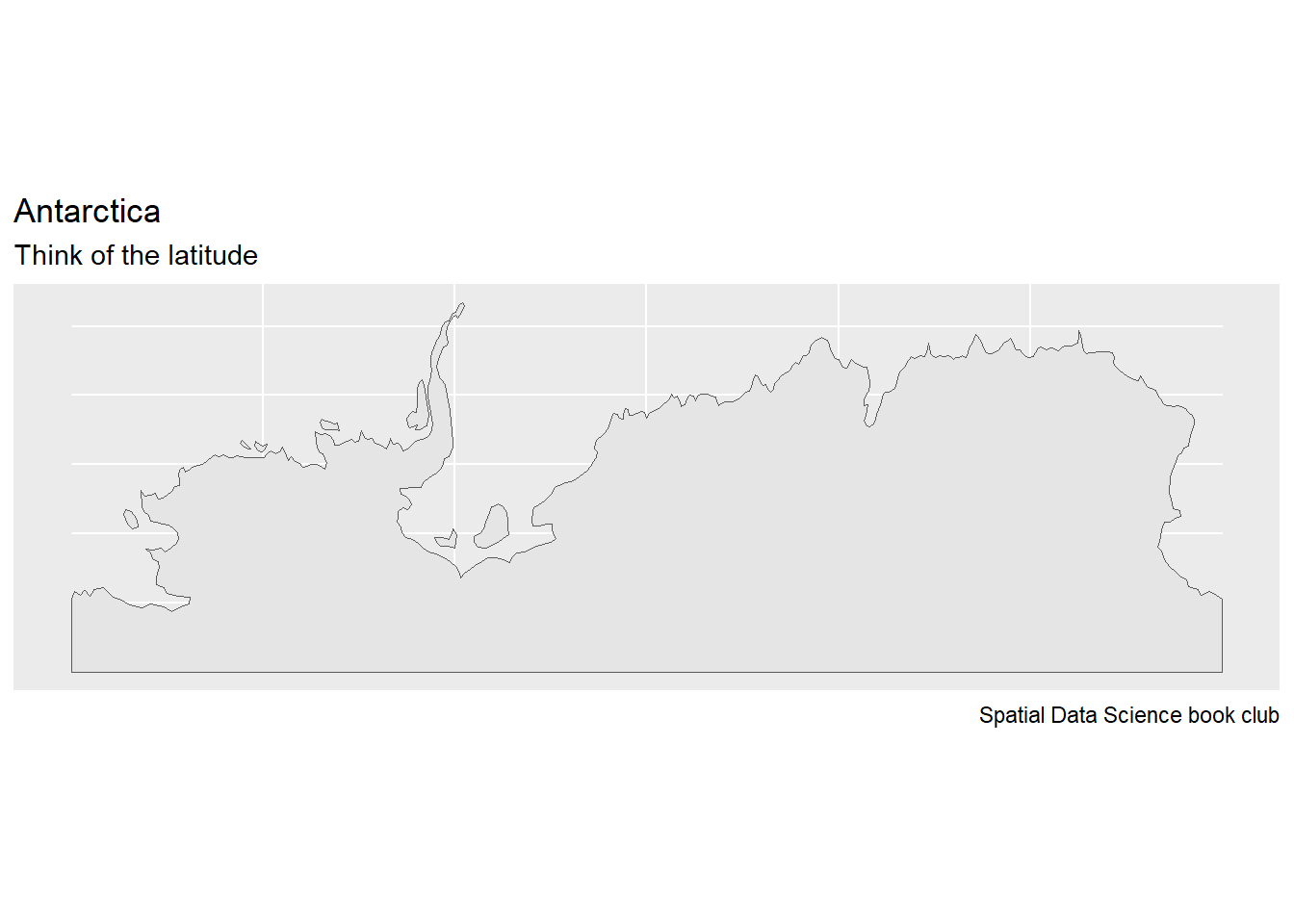sf::st_is_valid(Antarctica_map_B)
 TRUE

### Spherical (Polar Stereographic Projection)

Antarctica_map_C <- st_geometry(Antarctica_map_A) |>
st_transform(3031)
Antarctica_map_C |>
ggplot() +
geom_sf() +
labs(title = "Antarctica",
subtitle = "Think of the latitude",
caption = "Spatial Data Science book club")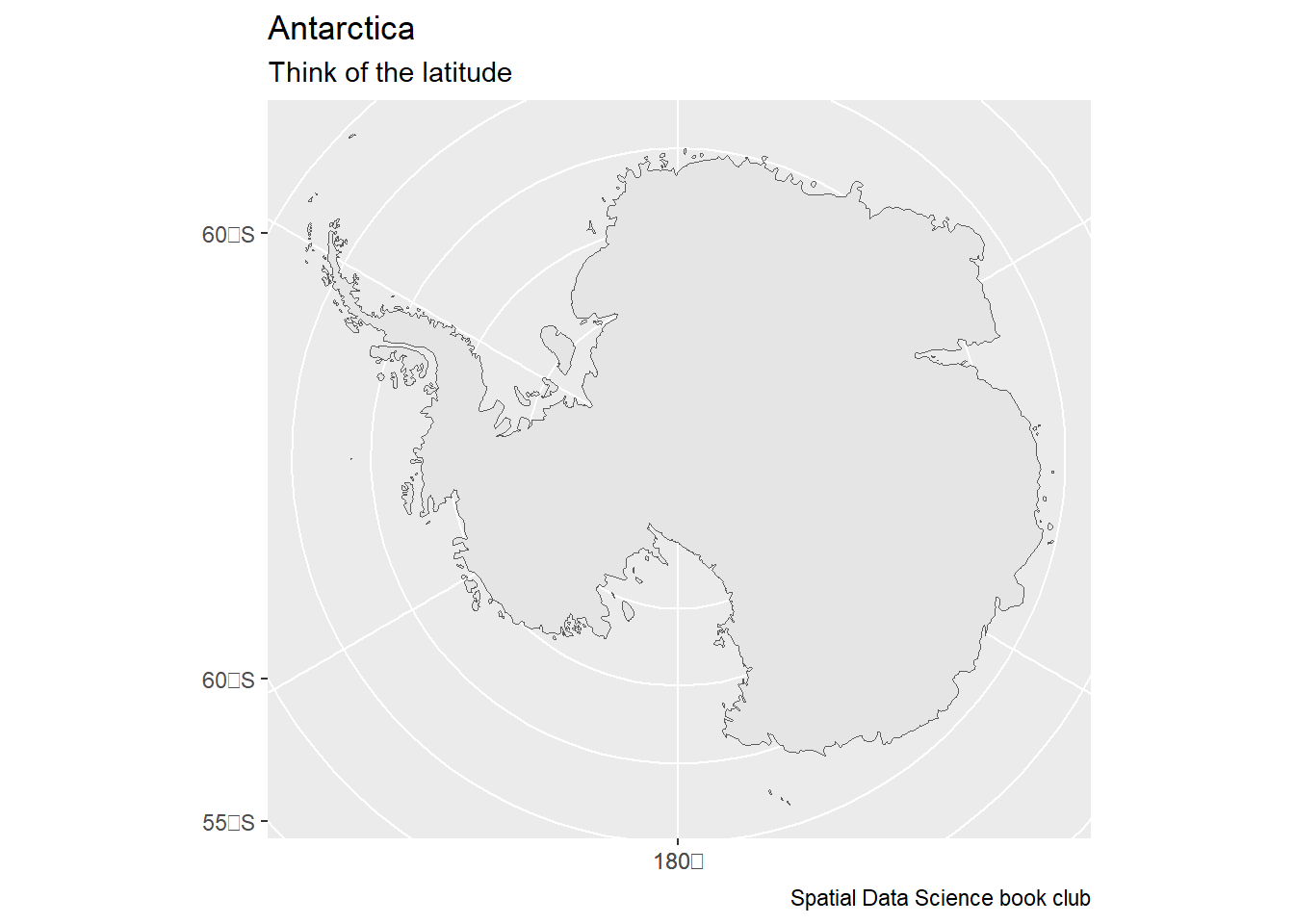sf::st_is_valid(Antarctica_map_C)
 TRUE
Antarctica_map_D <- st_geometry(Antarctica_map_B) |>
st_transform(3031)
Antarctica_map_D |>
ggplot() +
geom_sf() +
labs(title = "Antarctica",
subtitle = "Think of the latitude",
caption = "Spatial Data Science book club")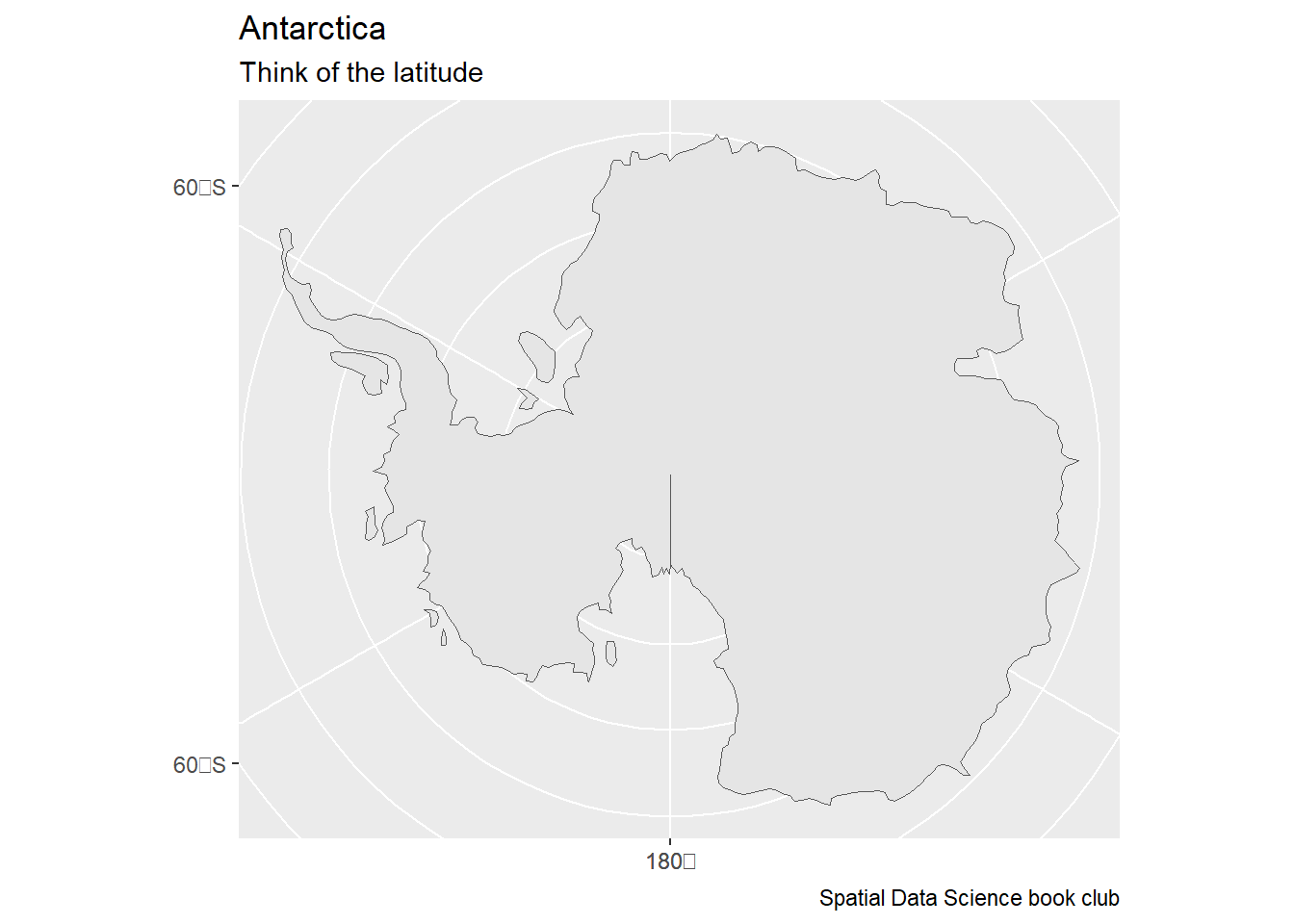sf::st_is_valid(Antarctica_map_D)
 TRUE

## Meeting Videos

### Cohort 1

Meeting chat log
LOG